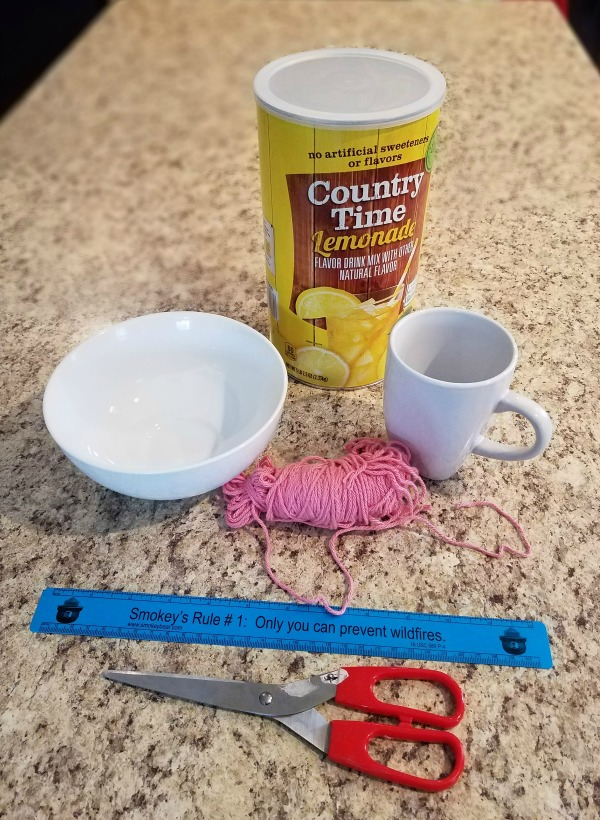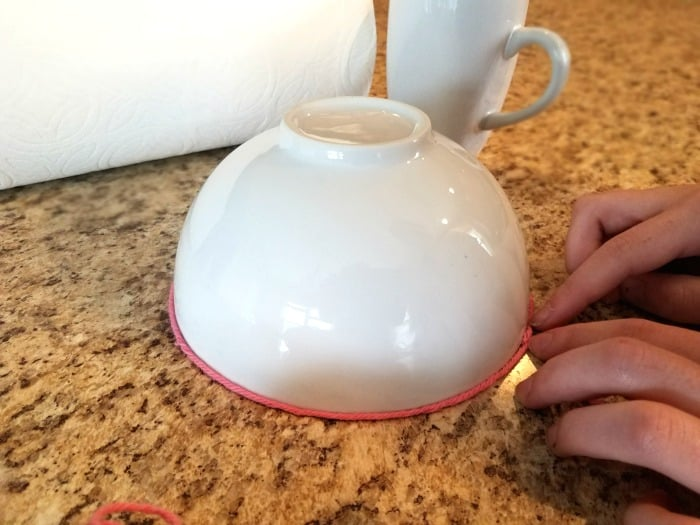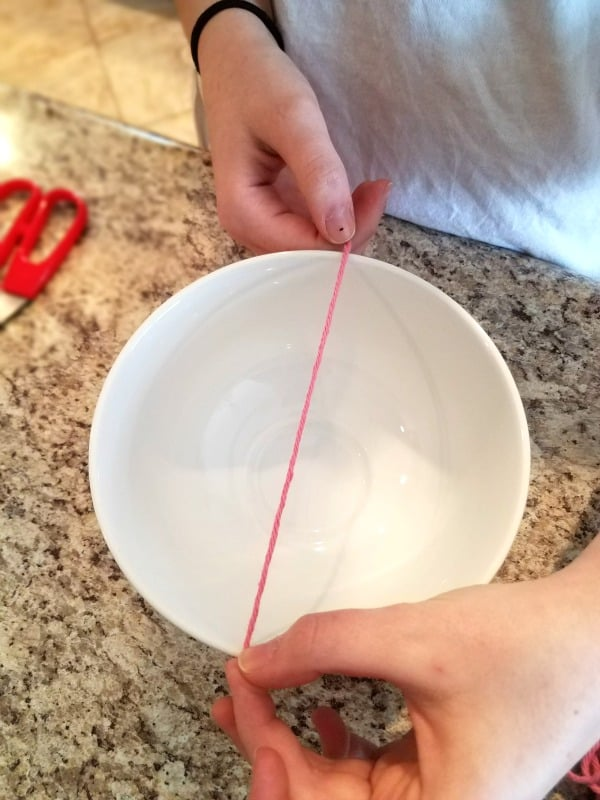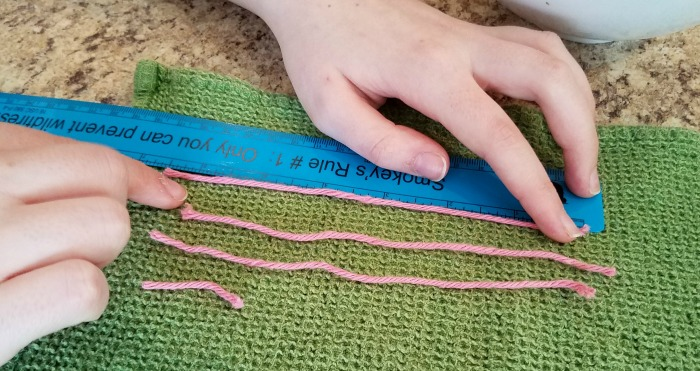Thursday March 23, 2023
Latest:
17 total views

Pi is a mathematical constant that demonstrates the relationship between the circumference and the diameter of a circle. What do I mean by that?## What Is Pi?

The circumference of a circle is the distance around the outside of the circle and the diameter is the distance across the center of the circle. If you take the circumference of a circle and divide it by its diameter, you get a number 3.1415926535897932….. This is true for all circles of any size. No matter what size the circle, when you divide it’s circumference by its diameter, you will get this same number. We call this number pi. So, we can see the mathematical formula for pi (π) is:
π= c/d

When you calculate pi by hand on paper or on a calculator, you see that it seems to go on forever. That’s because it does. Pi is an irrational number with no known end. It has an infinite number of digits. Just for fun, go to PiDay.org and check out the first million digits of Pi.

When we calculate irrational numbers, we choose how many digits we are going to use. For basic calculations, we can just say π = 3.14. However, we would use more digits of pi for more precise calculations.

Related post: Pi Activity – Make a Cityscape and Celebrate Pi Day!

## Demonstrating Pi

When we learn mathematical formulas like the one we use to calculate pi, we often take it for granted that these are true and correct. Sometimes, the math is just to complicated for us to prove at the time. The formula for pi is not like that. We can use simple measurements and basic math to demonstrate that the formula for pi is true.Supplies

• string or yarn
• circular objects
• scissors
• ruler or tape measure
• paper
• pencil

ProcedureWrap a length of string around a circular object. This might be a trash can, a coffee cup, or a basketball hoop, for examples. Cut the string the same length as the circumference around the circle.Take that string and stretch it across the diameter of the same circle. Cut a length of string the same length as the  diameter of the circle. Do this as many times as you can with the original string.In the end, you should have 3 pieces of string the same length as the diameter of the circle and  a fourth smaller piece. Measure the smaller piece and compare it to the length of the longer pieces. What fraction is the smaller piece to the larger piece?

Repeat this process with several other circular objects. How did the fraction of the smaller pieces relate?

If you cut and measured very carefully, you should get 3 diameter lengths of string and a smaller piece that should calculate to be around 0.14 of the diameter. Most calculations will not be exact, however discuss the ratio and significance of your findings.

## More Geometry and Math Posts

Pi Activity – Make A Cityscape

Christmas Geometry Project (could be used all year long)

Geometry Scavenger Hunt

Space Themed Math Challenge Printables

Using Cooking To Teach Math And Science {with printable}

What Sets Teaching Textbooks Homeschool Math Apart?

High School Math Resources

Fun Math Manipulatives For All Ages

Flash Card Math Games

Football Math

Math For Horse Lovers

### News

Recycling in your classroom can easily be made a daily activity. Try implementing one or more of these recycling…